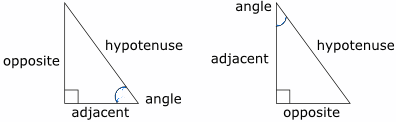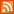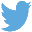Bucaro TecHelpHTTPS Encryption not required because no account numbers orpersonal information is ever requested or accepted by this siteCustom Search# Java Script Math Object Trigonometric Methods

Java Script Math.sin Method

Sine is a trigonometric function. Based on a right triangle. It's the ratio of the length of the opposite side to the length of the hypotenuse.

sine A = opposite/hypotenuseIn identifying the sides of a right triangle, the side next to the angle for which we are using the trigonometric function is called the adjacent side. The side opposite that angle is called the opposite side.

If you know an angle and the length of the side opposite the angle, you can use the sine of the angle to calculate the length of the hypotenuse.

length of hypotenuse = length of opposite/sine of angle

Example

```angle = 40 degrees
opposite side = 9
```

Java Script Using Math.sin Method

```<script type="text/javascript">

var angle = 40;
var opposite = 9;

var hypotenuse = opposite/Math.sin(40 * Math.PI/180);

</script>
```

The code shown above uses the Java Script Math.sin method. The Math.sin method requires the angle passed to it to be in radians. A radian is the angle of an arc created by wrapping the radius of a circle around its circumference. It sounds like a weard unit, by by coincidence there are 2 PI radians in a complete circle. This makes this much easier in fields like calculus. To convert degrees to radians, multiply by PI/180.

The code shown above also uses the Number objects .toFixed(n) method. This method converts a value to a number with n digits to the right of the decimal point.

Java Script Math.cos Method

Cosine is the ratio of the length of the adjacent side to the length of the hypotenuse.

If you know an angle and the length of the side adjacent to the angle, you can use the cosine of the angle to calculate the length of the hypotenuse.

length of hypotenuse = length of adjacent/cosine of angle

Example

```angle = 35 degrees
opposite side = 8
```

Java Script Using Math.cos Method

```<script type="text/javascript">

var angle = 35;
 .menubtn { margin-top:10px; margin-left:50px; width:220px; opacity:1; border-style:solid; border-color:#009000; background-color:#66ff66; transition-property: opacity; transition-duration: 1s; transition-timing-function: ease-out; } .menubtn:hover { opacity:1; background-color:#e5ff23; } Menu - More Java Script ProgrammingRSS FeedFollow @Stephen Bucaro